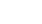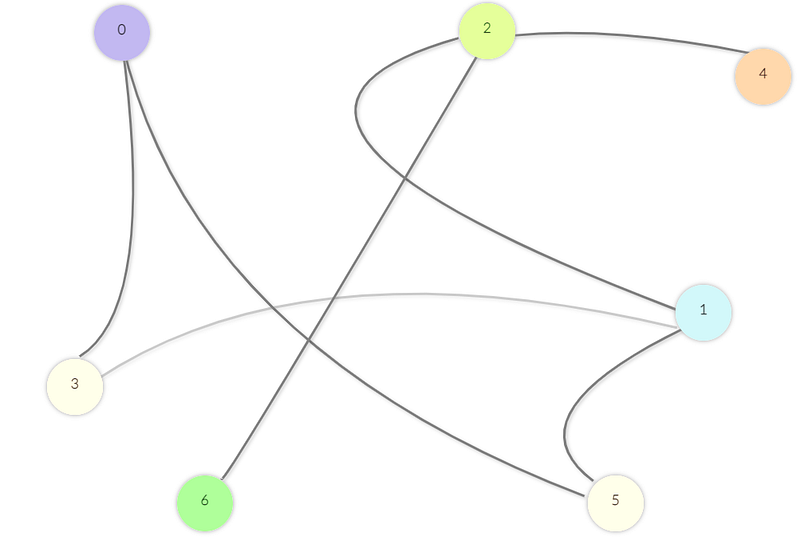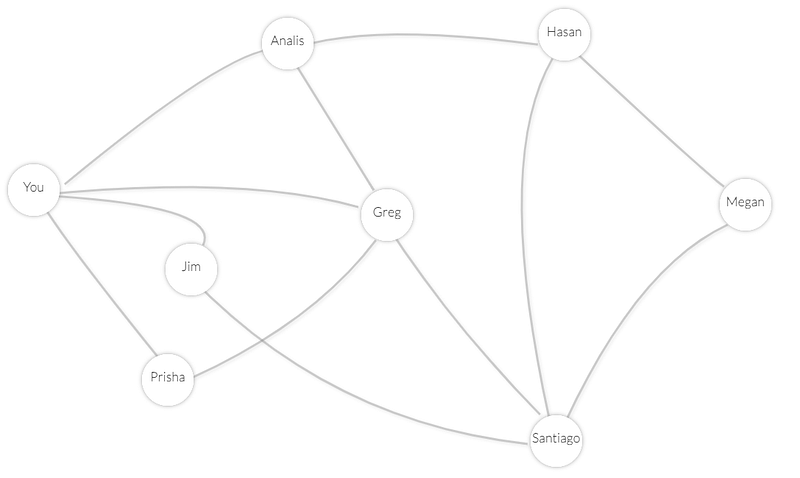# Shaking off the Rust### Febuary 19th, 2022Difficulty: Intermediate

Shaking off the Rust is a series of exercises with the Rust programing language. The purpose of the series is to improve both my and my dear reader's abilities with Rust by building things. Plus, by actually building stuff, we'll learn about an array of technological concepts in the process. In this installment, we're going to perform breadth-first search.

Rust is good. Breadth-first search is good. Good.

### Blazingly Fast Intro to Graphs

Whenever we're dealing with graph algorithms, the word graph refers to a mathematical structure that models a set of connections . Graphs consist of nodes and edges.

Let's say you raise alpacas, and you want to find your closest LinkedIn connection that's in the market for a handsome young alpaca.

Using a graph to model the situation, a node will represent a person, and an edge will represent a connection between two people. That could look like this:Suppose that Megan wants to buy an alpaca. We'll see how breadth-first search can help us discover Megan, and the connections that lead us to Megan.

### Blazingly Fast Intro to Breadth-First Search

The Breadth-First Search algorithm begins at a chosen node $x$﻿. It explores all of the nodes directly connected to $x$﻿. It then explores all the nodes that are two connections away from $x$﻿. Then three connections away, and so on until the search is complete . That is, it explores all nodes $d$﻿ edges away from $x$﻿ before exploring any nodes $d+1$﻿ edges away from $x$﻿; it explores nodes in layers .

1. Determine if there is a path from node $x$﻿ to node $y$﻿.

or

1. Find the shortest path from node $x$﻿ to node $y$﻿ .

Going back to our alpaca farm, let's visualize how breath-first search will find Megan, our closest potential customer (shown below is an applet I made to help us understand Breadth First Search, scroll to the bottom of this article to give it a whirl).

Before getting into the workings of breadth-first search and coding our Rust implementation, we'll need a blazingly fast introduction$^{\text{TM}}$﻿ to the queue data structure.

### Blazingly Fast Intro to Queues

Queues store a collection of data items, which are accessed in a first-in, first-out (FIFO) order . The queue supports at least two operations:

- Enqueue: insert an item into the back of the queue.

- Dequeue: remove and return an item from the front of the queue.

A queue is an integral piece of breadth-first search: the algorithm uses a queue to keep track of the nodes it has visited.

### Getting Started

We'll begin by creating a new library with Cargo.

cargo new bfs --lib
cd bfs

### Implementing a Queue

We'll use a struct to implement our queue.

// lib.rs

use std::collections::VecDeque;

struct Queue<T> {
pub items: VecDeque<T>,
}

impl<T> Queue<T> {
pub fn new() -> Queue<T> {
Queue {
items: VecDeque::new(),
}
}

pub fn enqueue(&mut self, v: T) {
self.items.push_back(v)
}

pub fn dequeue(&mut self) -> T {
self.items
.pop_front()
.expect("Cannot dequeue from empty queue.")
}

pub fn is_empty(&self) -> bool {
self.items.len() == 0
}
}

Since queues support storage of data independent of the type of data, we are implementing the Queue struct with a generic. Generics are stand-ins that allow us to write code that operates on multiple concrete types or other properties .

If you're unfamiliar with Rust's generic types, I suggest reading section 10.1 of the Rust programming language book. Generics are an essential component of a Rust programmer's toolkit.

VecDeque is a “double-ended queue implemented with a growable ring buffer” . This provides a $O(1)$﻿ enqueue/dequeue time (thank you to Reddit user svetlin_zarev for suggesting VecDeque).

The pop_front method returns an Option containing the first element of the VecDeque, or None if the VecDeque is empty.

In the Rust standard library, the Option enum represents a value that may or may not exist - an optional value. An instance of an Option is either None or Some. If it is Some, then it contains a value .

expect is an important method. If the Option it is operating on is None, expect panics with a custom error message. Otherwise, the contained Some value is returned .

The rest of our queue implementation seems self-explanatory to me, but please don't hesitate to email me at joshtaylor361@gmail.com if something doesn't make sense.

### Representing a Graph in Rust

We will use a collection of adjacency lists to represent a graph in our code. This collection of adjacency lists is a vector of size $|V|$﻿, where $|V|$﻿ is the number of nodes in the graph. Let $G$﻿ denote the collection of adjacency lists. For every list $v$﻿ in $G$﻿, $G[v]$﻿ will be a vector containing all vertices that $v$﻿ is connected to.

We can use Rust's custom types to help us implement our adjacency list representation.

// lib.rs

/*...*/

type Vertex = Vec<u32>;
type Graph = Vec<Vertex>;

Nice.

### O l'oun t'awa se n'yara Je k'abere

We'll use our alpaca example once again to help describe breadth-first search. The algorithm can be broken into six steps :

1. Initialize an empty queue $Q$﻿. This will eventually contain people we find on LinkedIn. Enqueue yourself.
1. Dequeue a person $p$﻿ from $Q$﻿.
1. Let $p_1...p_n$﻿ denote the people connected to $p$﻿. For each person $p_i$﻿ in $p_1...p_n$﻿, check if we've already asked $p_i$﻿ if they're interested in purchasing an alpaca. If we have already asked, skip to step 5.
1. Ask $p_i$﻿ if they want an alpaca. If so, mission accomplished - we can stop our search! Otherise, if $p_i$﻿ does not care to buy an alpaca, add $p_i$﻿ to $Q$﻿ and record that we have already asked them about our alpacas.
1. Loop back to step 2 until $Q$﻿ is empty.
1. If the queue is empty, then we have not found any potential customers and we will starve.

Here's pseudocode for the algorithm, which will be followed by a Rust implementation. Note that we're also recording the path from the start_node to the end_node, should that path exist. This is what the prev list is for, which records the order in which we visit nodes.

bfs arguments {
graph: an adjacency list representation of a graph,
start_node: integer,
end_node: integer
}

bfs(graph, start_node, end_node) returning path from start_node to end_node if path exists, otherwise empty list {
q = empty queue
q.enqueue(start_node)

visited_vertices = initialize list of false values of length |V|
visited_vertices = true

prev = initialize list of null values of length |V|

while queue is not empty {
current_node = queue.dequeue()
for each v in graph[current_node] {
if v == end_node {
prev[v] = current_node
break outer loop
}

if !visited_vertices[v] {
queue.enqueue(v)
visited_vertcies[v] = true
prev[v] = current_node
}
}
}

path = empty list
at = end_node

while at is not null {
path.push(at)
at = prev[at]
}

reverse(path)

if path == start_node {
return path
}

return empty list

}

And here's our Rust implementation:

//  lib.rs

/*...*/

fn bfs(graph: Graph, start_node: u32, end_node: u32) -> Option<Vec<Option<u32>>> {
let mut queue = Queue::new();
queue.enqueue(start_node);

let mut visisted_vertices = vec![false; graph.len()];
visisted_vertices = true;

let mut prev: Vec<Option<u32>> = vec![None; graph.len()];

'outer: while !queue.is_empty() {
let current_node = queue.dequeue();
for v in graph[current_node as usize].iter() {
if *v == end_node {
prev[*v as usize] = Some(current_node);
break 'outer;
}

if !visisted_vertices[*v as usize] {
queue.enqueue(*v);
visisted_vertices[*v as usize] = true;
prev[*v as usize] = Some(current_node);
}
}
}

let mut path = Vec::new();
let mut at = Some(end_node);
while at != None {
path.push(at);
at = prev[at.unwrap_or(0) as usize];
}

path.reverse();

return match path {
Some(x) if x == start_node => Some(path),
_ => None,
};
}

I'll go through the implementation, and highlight features of Rust that could use illumination. If I miss something you don't understand, again, feel free to email me at joshtaylor361@gmail.com.

• let mut visisted_vertices = vec![false; graph.len()]; - initializes a Vector of false values of length graph.len().
• let mut prev: Vec<Option<u32>> = vec![None; graph.len()]; - initializes a Vector of None values.
• 'outer: while !queue.is_empty() and break 'outer; - Rust allows us to break out of an outer loop from within a nested loop . This is accomplished by annotating our outer loop with a 'label and passing that label into the break statement. Note that outer is not a key word here - 'foo: while !queue.is_empty(), break 'foo; would work just as well.
• [*v as usize] - You can see a few points where we access an element of a vector with [*v as usize]. usize is a primitive type for pointer-sized unsigned integers. Its size is the number of bytes it takes to reference any location in memory . In Rust, vectors must be indexed by numbers of type usize. Thus, we perform the type conversion to usize.
• at = prev[at.unwrap_or(0) as usize]; - unwrap_or is a method on the Option enum. If the Option is Some, unwrap_or will return the contained Some value. If the the Option is None, unwrap_or returns the provided default, which is 0 in our case.
• return match path {
Some(x) if x == start_node => Some(path),
_ => None,
};

Recall that path is a vector of type Vec<Option<u32>>. This match expression checks if the first element in path is the start_node we provided. If so, the function will return the path. If the first element is either None or not start_node - meaning there is no path from start_node to end_node - then the function returns an empty vector.

### Testing

Here are two tests for our algorithm - one where there exists a path from start_node to end_node, and one where there does not.

// lib.rs

/*...*/

#[cfg(test)]
mod tests {
use super::*;

#[test]
fn existing_path() {
let G: Graph = vec![
vec![1, 2],
vec![0, 3, 4, 1],
vec![0, 4],
vec![1, 4, 5],
vec![1, 2, 3, 5],
vec![3, 4, 6],
vec![7, 5],
vec!,
];

assert_eq!(
bfs(G, 0, 7).unwrap(),
vec![Some(0), Some(1), Some(3), Some(5), Some(6), Some(7)]
)
}

#[test]
fn no_existing_path() {
let G: Graph = vec![
vec![1, 2, 5],
vec![0, 1, 3, 4],
vec![0, 3],
vec![1, 4, 5, 2],
vec![1, 3, 5],
vec![0, 3, 4, 1],
vec!,
vec!,
];

assert_eq!(bfs(G, 0, 7), None)
}
}

If running cargo test doesn't fail, then congratulations, you've implemented breadth-first search in Rust!

I've made an applet for playing around with breadth-first search. You may wish to test your understanding of graphs and breadth-first search by recreating the test cases in the applet.

In a future installment of SOTR, we'll see how to power this applet with WebAssembly.

Thanks for coding through another installment. I hope this post has left you one step closer to becoming the best programmer you can be.

### References

 Rust by Example.

 Rust Documentation on usize.

 Rust Documentation on VecDeque.

 Rust Documentation on expect.

## Support Me

Creating and running Shaking off the Rust is one of the most fulfilling things I do. But it's exhausting. By supporting me, even if it's just a dollar, you'll allow me to put more time into building this series. I really appreciate any support.

The only way to support me right now is by sponsoring me on Github. I'll probably also set up Patreon and Donorbox pages soon.

Thank you so much!